# An Introduction to Humidity and the Physics of Water Vapor

Humidity describes the amount of water vapor in the air. Water vapor is the gaseous state of water and is invisible. A number of ways are available to express humidity, such as absolute humidity, relative humidity, mixing ratio and dew point temperature. This article discusses the origin of water vapor in air and describes the most commonly used definitions for humidity.

## Origin of Water Vapor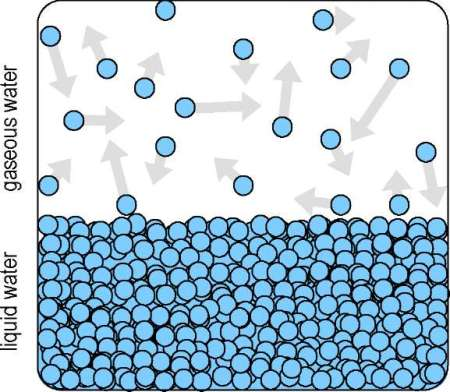Figure 1. Closed chamber with liquid water and gaseous water particles (vapor).

Let us consider a closed chamber with liquid water, as illustrated in Figure 1. The temperature governs the average kinetic energy, and therefore the impulse of the water particles in the liquid water. Certain particles exhibit higher energy and can exit from the liquid (evaporation). After a period to time, equilibrium occurs at a point where the number of water particles exiting the surface of the liquid water is same as the number of particles rejoining it. When this equilibrium state is attained, the number of gaseous water particles remains constant.

## Saturated Vapor Pressure

The gaseous water particles bombard the wall of the closed chamber, applying pressure (e) on the wall. In the equilibrium state, this pressure is known as saturated vapor pressure ei above ice or ew above water.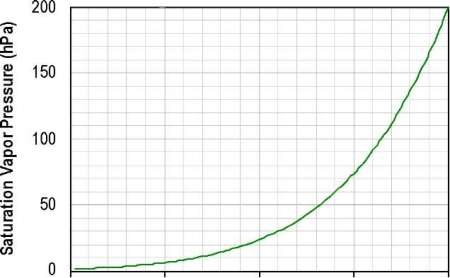Figure 2. Saturated Vapour Pressure ew(t)

Figure 2 shows the saturated vapor pressure above water. The related saturated vapor pressure above ice ei (t) is determined according to the Magnus formula, as expressed below, using the relating parameters from Table 1.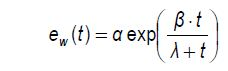where t is the temperature in °C and parameters á, â, and ã are given in Table 1.

Table 1. Parameters for equation 1

Condition T range (°C) á (hPa) â ã (°C)
Above water -45 – 60 6.112 17.62 243.12
Above ice -80 – 0.01 6.112 22.46 272.62

Between temperatures of -45°C and 60°C, the Magnus formula has an uncertainty of below ±0.6% at 95% confidence level. When more precision is needed, equations for saturated vapor pressure usually result in absolute functions that can only be solved numerically.

## Water Vapor Enhancement Factor

Additional gas mixtures or gases are often present in air. In such a case, the absolute barometric pressure is the sum of the partial pressures.

p = pnitrogen + poxygen + pothers+ e

Since there is interaction between different gas particles in air, a pressure dependent correction may be needed to change the water vapor pressure. The actual saturated vapor pressure above ice e’i or water e’w is given by

e'w = ew ⋅ fw or e'i = ei ⋅ fi

where the water vapor enhancement factor f is given by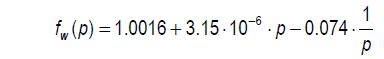and the barometric pressure p can vary from 3kPa to 110kPa. At standard barometric pressure, it can be assumed that f = 1. At 6bar, 1.02 is the enhancement factor.

## Terms of Humidity

As described above, terms of humidity include relative humidity, absolute humidity, dew point temperature, mixing ratio, and Heat Index.

## Relative Humidity

Relative humidity is the ratio of the partial vapor pressure in air e to the saturated vapor pressure at a given temperature t, ew (t). Relative humidity above ice Ui or above water Uw is mostly utilized for measurement purposes.

## Dew Point Temperature

The dew point temperature td is the temperature to which a quantity of air is cooled down such that condensation occurs at constant pressure.

## Absolute humidity

Absolute humidity is the mass of water vapor mH2O per humid air volume V and can be given as

dv = mH2O/V

## Mixing Ratio

Mixing Ratio is defined as the ratio of the mass of dry air and the mass of water vapor. It is expressed in terms of relative temperature and humidity using equation (1).

## Heat Index

Heat Index indicates how the human body experiences temperature. When relative humidity is high, the evaporation rate from the human skin is reduced. In such a case, heat cannot be dissipated from the body as easily as in the case of dry air. When there is low relative humidity, human body cools itself by perspiration and dissipates heat.

Based on subjective measurements, the Heat Index is only meaningful above 40%RH and 25°C. The Heat Index in °C is given by

HI = c00 + c10 t + c01 Uw + c11 tUw+ c20 t2 + c02U2w + c21t2Uw + c12tU2w + c22t2U2w

Table 2. Coefficients for Heat Index formula.

 c00 -8.7847 c10 1.6114 c01 2.3385 c11 -0.1461 c20 -0.0123 c02 -0.0164 c21 2.2117•10-3 c12 7.2546•10-4 c22 -3.5820•10-6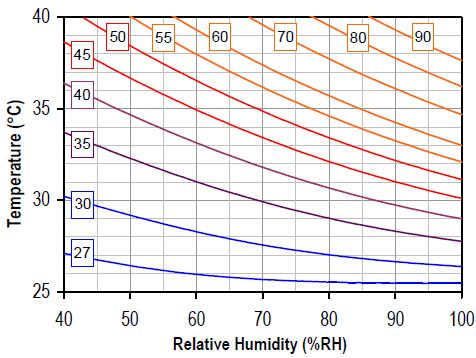Figure 3. Heat Index in °C.

The Heat Index as a virtue of relative temperature and humidity is shown in Figure 3. The meaning of the values is defined as follows:

• < 30°C: no discomfort
• 30 – 40°C: some discomfort
• 40 – 45°C: great discomfort
• > 45°C: dangerous
• > 54°C: heat stroke imminent

## Psychrometric Chart

Psychrometric charts, also known as Mollier diagrams, show aequi-potential curves of enthalpy, corresponding relative humidity on charts of temperature and mixing ratio. They aid in navigating systems where temperature and humidity play a major role, for example a tumble dryer.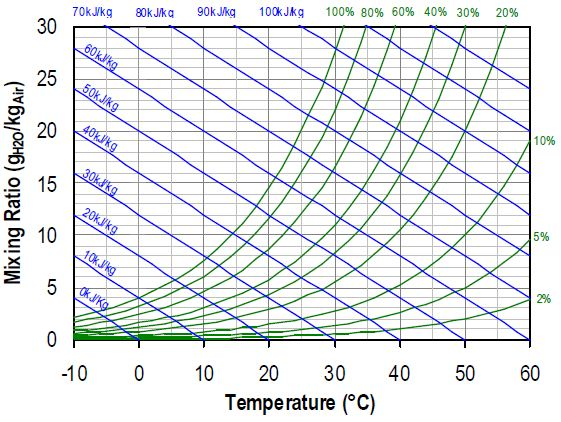Figure 4. Mollier Diagram: Blue lines are of equal enthalpy, red lines denote equal relative humidity.

Figure 4 shows a standard Mollier diagram with Aequi-potential curves of enthalpy (blue lines) and curves of equal relative humidity (green lines).

## Wet- and Dry-Bulb Temperature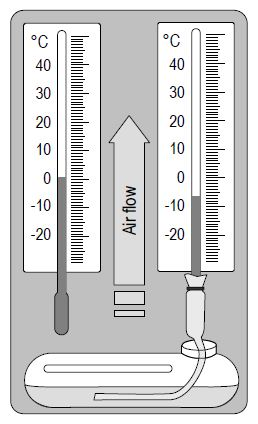Figure 5. Sketch of a wet bulb hygrometer or psychrometer.

Figure 5 displays a wet and dry bulb hygrometer, also called as psychrometer. The wet bulb thermometer calculates lower temperature than the dry bulb thermometer due to evaporation at its bulb. Evaporation depends on the local relative humidity. One of the thermometers is enclosed in a porous medium, which is kept wet by a wet sock. The temperature of the wet sock reduces as it evaporates water. Since the evaporation is stronger in dry air when compared to wet air, the difference in temperature is a measure for relative humidity.

## Conclusion

The article discusses the physics of humidity and describes the most important definitions. Starting from relative temperature and humidity, it provides some physical framework and helps calculate most physical values describing water vapor.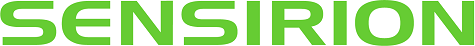This information has been sourced, reviewed and adapted from materials provided by Sensirion Inc.

## Citations

• APA

Sensirion Inc. (2020, September 14). An Introduction to Humidity and the Physics of Water Vapor. AZoSensors. Retrieved on October 28, 2020 from https://www.azosensors.com/article.aspx?ArticleID=488.

• MLA

Sensirion Inc. "An Introduction to Humidity and the Physics of Water Vapor". AZoSensors. 28 October 2020. <https://www.azosensors.com/article.aspx?ArticleID=488>.

• Chicago

Sensirion Inc. "An Introduction to Humidity and the Physics of Water Vapor". AZoSensors. https://www.azosensors.com/article.aspx?ArticleID=488. (accessed October 28, 2020).

• Harvard

Sensirion Inc. 2020. An Introduction to Humidity and the Physics of Water Vapor. AZoSensors, viewed 28 October 2020, https://www.azosensors.com/article.aspx?ArticleID=488.